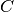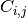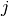This documentation is for scikit-learn version 0.16.1Other versions

If you use the software, please consider citing scikit-learn.

# sklearn.metrics.confusion_matrix¶

sklearn.metrics.confusion_matrix(y_true, y_pred, labels=None)[source]

Compute confusion matrix to evaluate the accuracy of a classification

By definition a confusion matrixis such thatis equal to the number of observations known to be in groupbut predicted to be in group.

Parameters: y_true : array, shape = [n_samples] Ground truth (correct) target values. y_pred : array, shape = [n_samples] Estimated targets as returned by a classifier. labels : array, shape = [n_classes], optional List of labels to index the matrix. This may be used to reorder or select a subset of labels. If none is given, those that appear at least once in y_true or y_pred are used in sorted order. C : array, shape = [n_classes, n_classes] Confusion matrix

References

Examples

```>>> from sklearn.metrics import confusion_matrix
>>> y_true = [2, 0, 2, 2, 0, 1]
>>> y_pred = [0, 0, 2, 2, 0, 2]
>>> confusion_matrix(y_true, y_pred)
array([[2, 0, 0],
[0, 0, 1],
[1, 0, 2]])
```